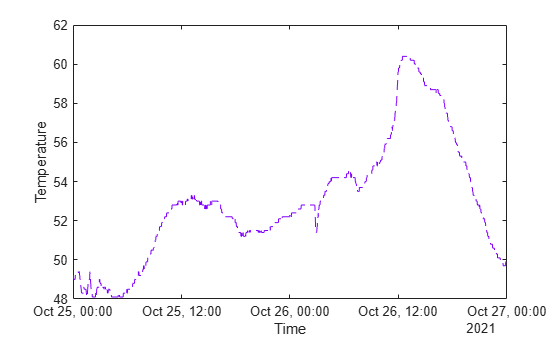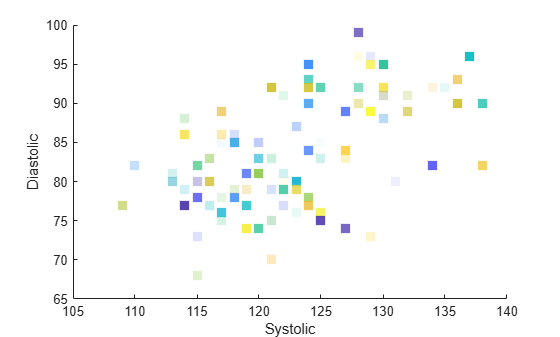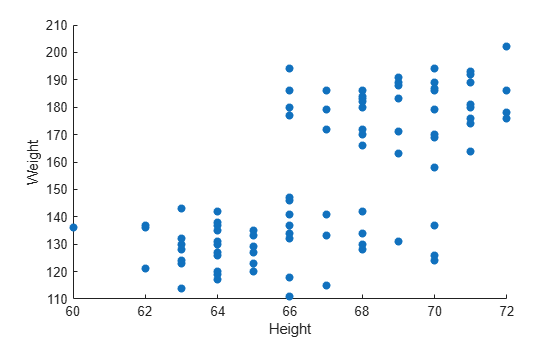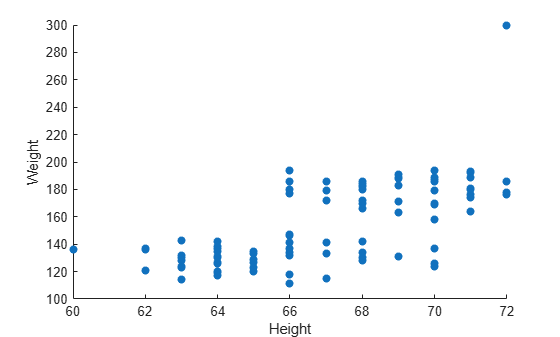## Plots That Support Tables

Many plotting functions can plot data directly from a table. You pass the table as the first argument to the function followed by the variables you want to plot. You can specify either a table or a timetable, and in many cases, you can plot multiple data sets together in the same axes.

The following examples use the `plot` and `scatter` functions to demonstrate the overall approach for plotting data from a table. To learn if a specific plotting function supports tables, refer to the documentation for that function.

### Create Simple Line Plots

Create a table containing three variables. Then pass the table as the first argument to the `plot` function followed by the names of the variables you want to plot. In this case, plot the `Input` variable on the x-axis and the `Output1` variable on the y-axis. Notice that the axis labels match the variable names.

```% Create a table Input = linspace(0,12)'; Output1 = sin(Input); Output2 = sin(Input/3); tbl = table(Input,Output1,Output2); % Plot the table variables plot(tbl,"Input","Output1")```To plot multiple data sets together, specify a string vector of table variable names for the x-coordinates, y-coordinates, or both. For example, plot the `Output1` and `Output2` variables together on the y-axis.

Because the y-coordinates come from two different table variables, it is not clear what the y-axis label should be, so the axis label remains blank. However, if you add a legend, the legend entries match the corresponding variable names.

```plot(tbl,"Input",["Output1","Output2"]) legend```### Customize Line Plots

To customize the appearance of lines after plotting with a table, set the `LineStyle` and `Color` properties. For example, read `weather.csv` as a timetable and plot the `Temperature` variable against the row times. Return the `Line` object as `p` so you can set its properties later.

Note: This code omits the variable for the x-coordinates. When you omit the x-coordinates, the y-coordinates are plotted against the row indices (for tables) or the row times (for timetables).

```tbl = readtimetable("weather.csv"); p = plot(tbl,"Temperature");```

Change the style of the line to dashed, and change the color to a shade of purple.

```p.LineStyle = "--"; p.Color = [0.5 0 1];```### Customize Scatter Plots

You can customize the appearance of the markers in scatter plots by setting properties after plotting with a table. For example, read `patients.xls` as a table and plot the `Diastolic` variable against the `Systolic` variable with filled markers. Return the `Scatter` object as `s` so you can set its properties later.

```tbl = readtable("patients.xls"); s = scatter(tbl,"Systolic","Diastolic","filled");```

Change the marker symbol to a square, fill the markers with a shade of light blue, and change the marker size to `80`.

```s.Marker = "sq"; s.MarkerFaceColor = [0.5 0.7 1]; s.SizeData = 80;```You can also vary the color and transparency of the markers according to table variables. For example, vary the colors according to the `Age` variable by setting the `MarkerFaceColor` property to `"flat"` and then setting the `ColorVariable` property to `"Age"`.

Vary the transparency according to the `Weigh`t variable by setting the `MarkerFaceAlpha` property to `"flat"` and then setting the `AlphaVariable` property to `"Weight"`.

```% Vary the colors s.MarkerFaceColor = "flat"; s.ColorVariable = "Age"; % Vary the transparency s.MarkerFaceAlpha = "flat"; s.AlphaVariable = "Weight";```### Update Plot by Modifying the Table

When you pass a table to a plotting function, a copy of the table is stored in the `SourceTable` property of the plot object. If you change the contents of the table stored in that property, the plot automatically updates to show the changes. (However, if you make changes to the table in your workspace, those changes have no effect on your plot.)

For example, read `patients.xls` as a table and plot the `Weight` variable versus the `Height` variable. Return the `Scatter` object as `s`, so you can access its properties later.

```tbl = readtable("patients.xls"); s = scatter(tbl,"Height","Weight","filled");```To change a value in the table, use dot notation to reference the table from the `SourceTable` property of the `Scatter` object. In this case, find the maximum value of the `Weight` variable and change it to `300`. The plot automatically updates.

```[~,idx] = max(s.SourceTable.Weight); s.SourceTable.Weight(idx) = 300;```### Combine Table and Vector Data

Many plots that support tables allow you to specify some aspects of your plot using a table variable and other aspects using vectors or matrices. For instance, you can create a scatter plot using coordinates from a table and customize the colors of the markers by setting the `CData` property to a vector, an RGB triplet, or a matrix of RGB triplets.

For example, create a scatter plot using data from a table. Read `patients.xls` as a table, and plot the `Weight` variable versus the `Height` variable.

```tbl = readtable("patients.xls"); s = scatter(tbl,"Height","Weight","filled");```Next, change the colors of the plotted points using a vector. When you combine data from different sources like this, the size of each vector, matrix, or table variable must be compatible with the plot you are creating. In this case, create a vector called `bpratio` by dividing the systolic values by the diastolic values from the table. Because `bpratio` is derived from the same table as the `Height` and `Weight` variables, it has the same number of elements as those variables, and so it is compatible with this plot.

Color each point according to the blood pressure ratio by setting the `CData` property to `bpratio`. Then add a colorbar.

```% Vary the color by blood pressure ratio bpratio = tbl.Systolic./tbl.Diastolic; s.CData = bpratio; % Add a colorbar colorbar```You can also plot vectors or matrices, and modify the plot using table variables. After you create the plot, set the `SourceTable` property, and then set the table-related properties that you want. Table-related properties typically have the word `Variable` in their names. For example, plot two vectors of 100 random numbers.

```x = rand(100,1); y = rand(100,1); s = scatter(x,y,"filled");```Change the marker colors so that they vary according to the values in a table variable. Read `patients.xls` as the table `tbl`. Set the `SourceTable` property and vary the marker colors according to the `Age` variable in the table. Because the table has 100 rows, and the plot has 100 points, the `Age` variable is compatible with the plot. Then, add a colorbar to the plot.

```% Set source table and vary color by age s.SourceTable = tbl; s.ColorVariable = "Age"; % Add a colorbar colorbar```Note: Standalone visualizations such as `heatmap` do not support combinations of table and vector data.